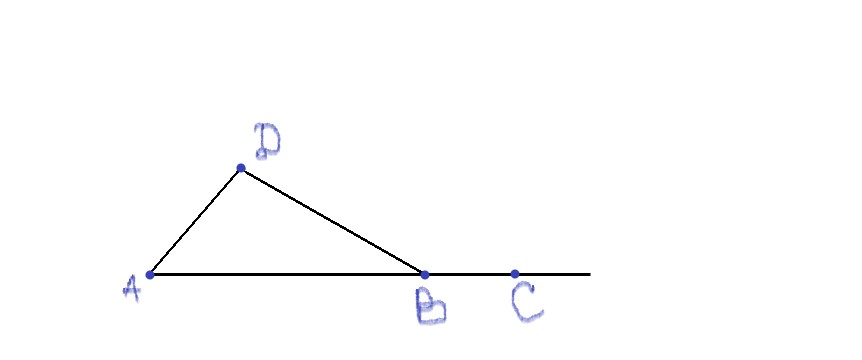2022-07-22

Proof of the Exterior Angle Theorem.
The exterior angle theorem for triangles states that the sum of “The measure of an exterior angle of a triangle is equal to the sum of the measures of the two interior angles that are not adjacent to it; this is the exterior angle theorem. The sum of the measures of the three exterior angles (one for each vertex) of any triangle is 360 degrees.” How can we prove this theorem?So, for the triangle above, we need to prove why $\mathrm{\angle }CBD=\mathrm{\angle }BAC+\mathrm{\angle }BCA$Brienueentismvh

Expert

Step 1
In the $\mathrm{△}ABC$, we know that ABD is a straight line. So $\mathrm{\angle }ABC={180}^{\circ }-\mathrm{\angle }CBD$. From the angle sum property of triangles we can infer that $\mathrm{\angle }BAC+\mathrm{\angle }ABC+\mathrm{\angle }BCA={180}^{\circ }$ or $\mathrm{\angle }ABC={180}^{\circ }-\left(\mathrm{\angle }BAC+\mathrm{\angle }BCA\right)$.
Step 2
Therefore: $\mathrm{\angle }ABC={180}^{\circ }-\mathrm{\angle }CBD={180}^{\circ }-\left(\mathrm{\angle }BAC+\mathrm{\angle }BCA\right)$ $⇒-\mathrm{\angle }CBD=-\left(\mathrm{\angle }BAC+\mathrm{\angle }BCA\right)$ $⇒-\mathrm{\angle }CBD×-1=-\left(\mathrm{\angle }BAC+\mathrm{\angle }BCA\right)×-1$ $⇒\mathrm{\angle }CBD=\mathrm{\angle }BAC+\mathrm{\angle }BCA$Ciara Rose

Expert

Step 1
Euclid gives a visually satisfying proof of the exterior angle theorem by drawing BE parallel to AC, and observing that $\mathrm{\angle }CBE=\mathrm{\angle }ACB$ (alternate interior angles) and $\mathrm{\angle }EBD=\mathrm{\angle }CAB$ (corresponding angles), making $\mathrm{\angle }CBD=\mathrm{\angle }ACB+\mathrm{\angle }CAB$. This theorem includes the further important result that the three angles of a triangle sum to ${180}^{o}$, or "two right angles" as Euclid says.Step 2
But if, as I suspect, the true intent of OP's question is, assuming the truth of the exterior angle theorem, prove that the sum of the three exterior angles of a triangle is ${360}^{o}$, then we can argue as follows.Since by the exterior angle theorem $\mathrm{\angle }CBD=\mathrm{\angle }BCA+\mathrm{\angle }BAC$ and $\mathrm{\angle }ACE=\mathrm{\angle }CBA+\mathrm{\angle }BAC$ and $\mathrm{\angle }BAF=\mathrm{\angle }CBA+\mathrm{\angle }BCA$ then by addition $\mathrm{\angle }CBD+\mathrm{\angle }ACE+\mathrm{\angle }BAF=2\mathrm{\angle }BCA+2\mathrm{\angle }CBA+2\mathrm{\angle }BAC=2\cdot {180}^{o}={360}^{o}$.

Do you have a similar question?